### Shape Comparison and Gromov-Hausdorff Distance

###### Sushovan "Sush" MAJHI
Tulane University (January 21, 2020)Scan the above QR or hit the following url: smajhi.com/gh-presentation

### The Manifesto

• Introduction to Shape Comparison
• Hausdorff Distance
• Gromov-Hausdorff Distance
• Computing the Gromov-Hausdorff Distance
• Questions, Discussions, and 🍕 Pizza.

### CollaboratorsCarola Wenk
Computer Science, TulaneJeffrey Vitter
Computer and Information Science, University of Mississippi

### Motivation

1. Distinguish Shapes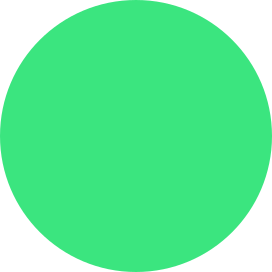circle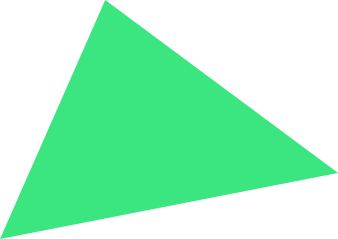triangle
2. Classify Shapestriangularcircular
3. Quality Guarantee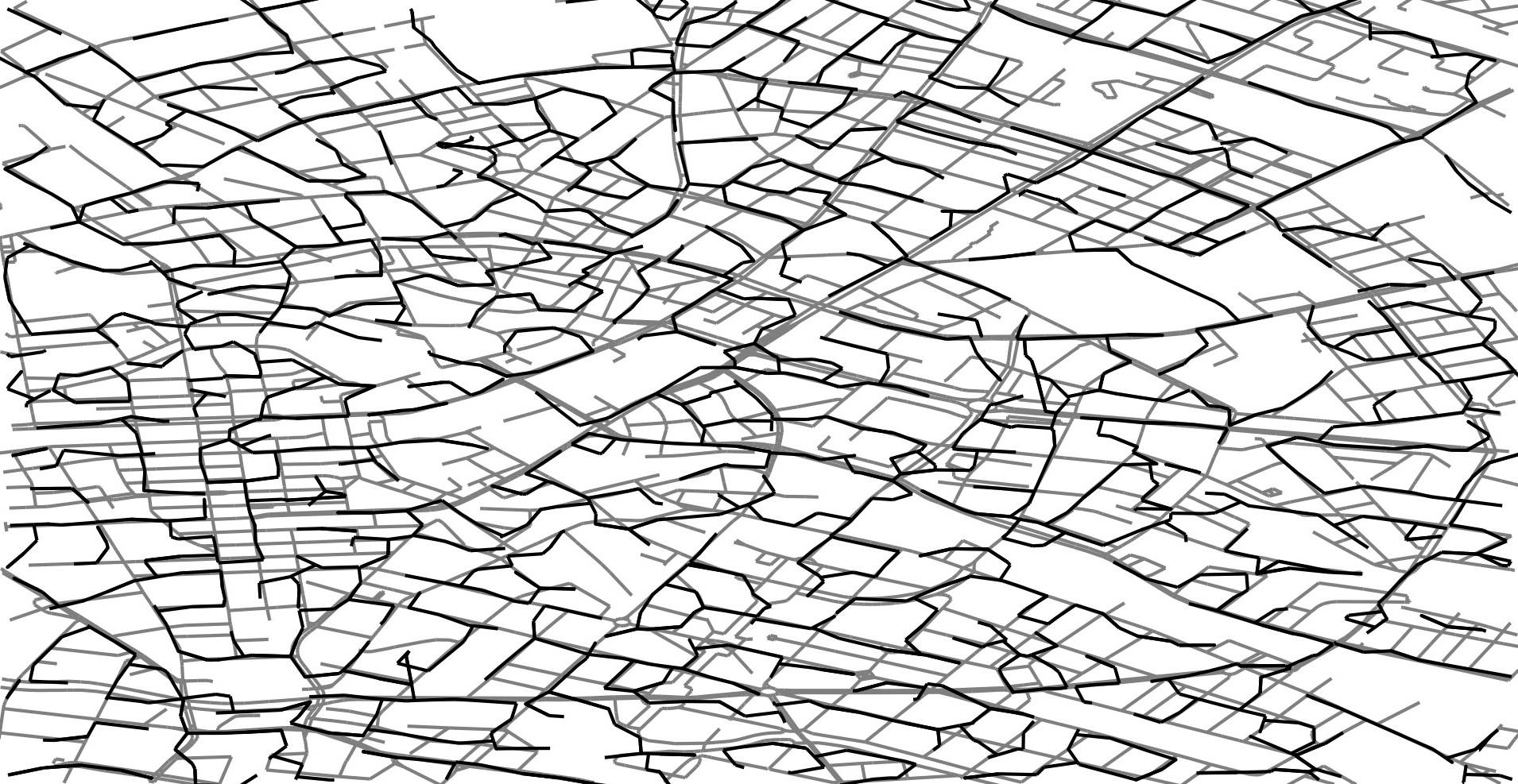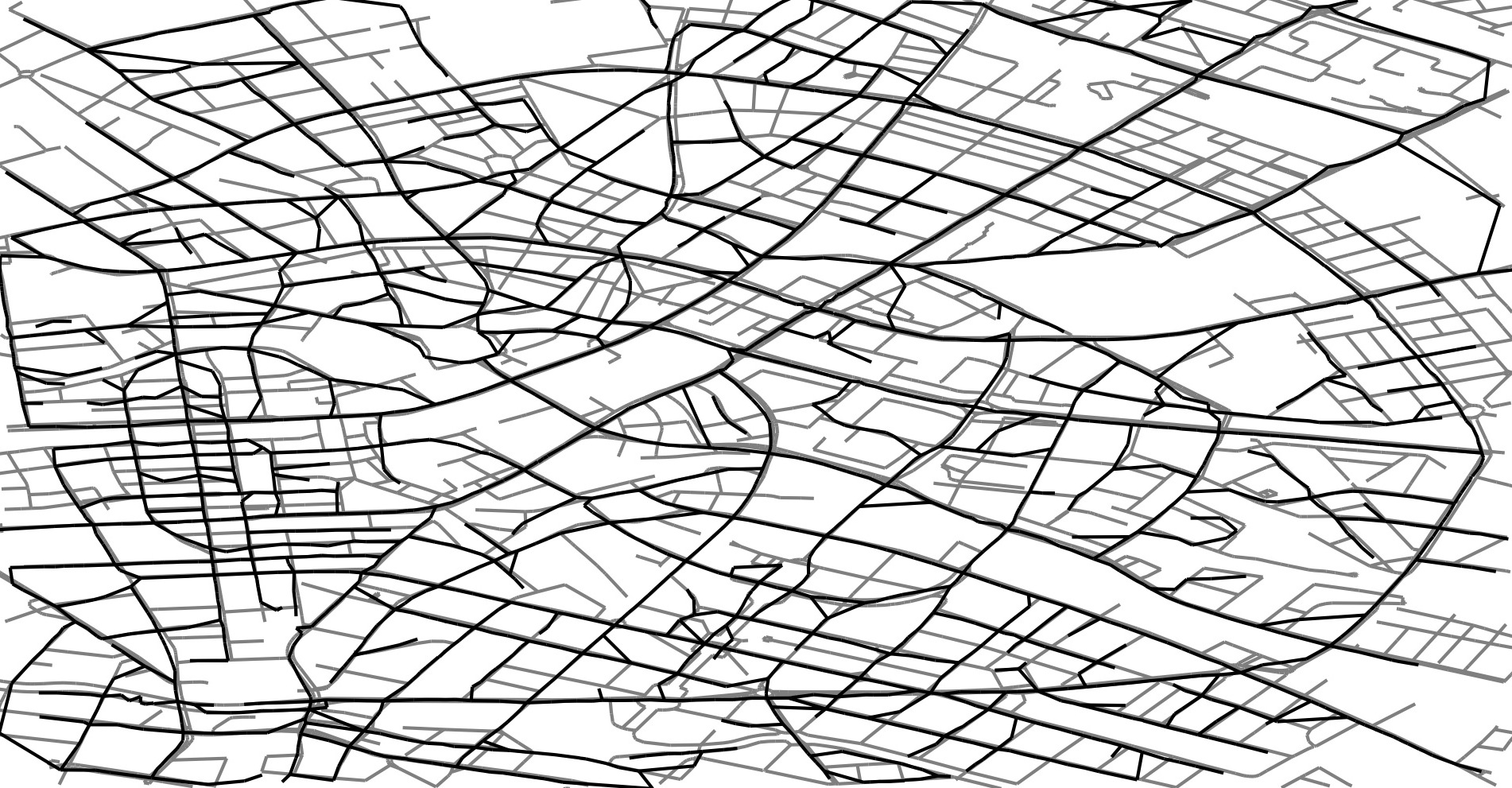Map Construction from GPS data (Berlin)
https://mapconstruction.org

### Shape Comparison

A shape can be modeled as a metric space $(X,d_X)$.

We need an appropriate notion of a distance measure $d_?(X,Y)$ so that

• $d_?(X,Y)$ defines a pseudo-metric on the class of metric spaces.

• $d_?(X,Y)$ large $\iff$ very different shape

• $d_?(X,Y)$ small $\iff$ $X=Y$ up to a class of deformation.

### Hausdorff Distance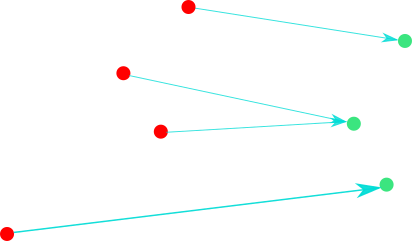Nearest neighbor distance (red points $\to$ green points).

Let $A,B\subseteq\mathbb{R}^N$. The directed Hausdorff distance from $A$ to $B$ is defined by $$\overrightarrow{d}_H(A,B)=\sup_{a\in A}\inf_{b\in B}\|a-b\|.$$
For any two compact $X,Y\subset\mathbb{R}^N$, $$d_H(X,Y)=\max\left\{\overrightarrow{d}_H(X,Y),\overrightarrow{d}_H(Y,X)\right\}$$
For any two compact $X,Y\subset(Z,d_Z)$, $$d^Z_H(X,Y)=\max\left\{\overrightarrow{d}^Z_H(X,Y),\overrightarrow{d}^Z_H(Y,X)\right\}$$
1. $d^Z_H(X,Y)\geq0$,

2. $d^Z_H(X,Y)=d^Z_H(Y,X)$,

3. $d^Z_H(X,Y)=0\iff X=Y$, and

4. $d^Z_H(A,C)\leq d^Z_H(A,B)+d^Z_H(B,C)$.
The Hausdorff distance induces a complete metric space on the set of all compact subsets of a metric space.

💡

$d_H$ is sensitive to Shape + Size + Placement.

### Hausdorff under Isometry

$d_H($◤, ◢ $)=$ large.

Let $\mathcal{E}(N)$ denote the class of Euclidean isometries or distance preserving maps $T:\mathbb{R}^N\to\mathbb{R}^N$ i.e. $\|T(a)-T(b)\|=\|a-b\|$.

1. For $N=1$, $T$ is translation or reflection.

2. For $N=2$, $T$ is rotation, translation or reflection.
For $X,Y\subseteq\mathbb{R}^N$, we define $$d_{H,iso}(X,Y)=\inf\limits_{T\in\mathcal{E}(N)}d_H\left(X,T(Y)\right)$$
💡

$d_{H,iso}$ is sensitive to Shape + Size + Placement.
$d_{H,iso}($◤, ◢ $)=0$.

### Gromov-Hausdorff Distance

How to compare shapes that do not have a common embedding?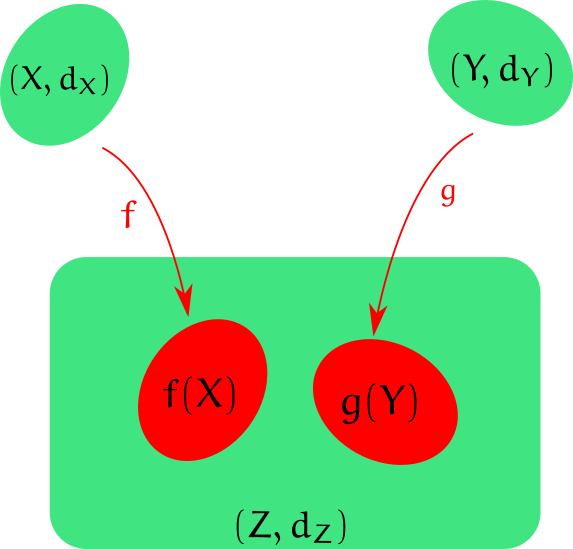Isometric Embedding

For two abstract metric spaces $(X,d_X)$ and $(Y,d_Y)$, we define $$d_{GH}(X,Y)=\inf\limits_{f,g,Z} d^Z_H(f(X),g(Y)).$$

### $d_{GH}$ vs $d_{H,iso}$

Let $X,Y\subseteq(\mathbb{R}^N,\|\cdot\|)$ be compact. Then, both $d_{GH}(X,Y)$ and $d_{H,iso}(X,Y)$ are defined. $$d_{GH}(X,Y)\leq d_{H,iso}(X,Y)\leq c_N\sqrt{Md_{GH}(X,Y)},$$ where $M=\max\{diam(X),diam(Y)\}$.
For $X,Y\subseteq\mathbb{R}^1$, we have $$d_{GH}(X,Y)\leq d_{H,iso}(X,Y)\leq\left(1+\frac{1}{4}\right)d_{GH}(X,Y),$$ and the upper bound is tight.

### Computing $d_{GH}$

For $|X|,|Y|\leq k$, computing $d_{GH}(X,Y)$ takes $O(2^k)$ time.
For $X,Y\subset\mathbb{R}^1$ and $|X|,|Y|\leq k$, computing $d_{H,iso}(X,Y)$ takes $O(k\log{k})$ time.
Sometimes, computing the exact optimal solution ($s_{opt}$) to an optimization problem is HARD.

💡

Computing an approximate solution ($s_{appx}$) is sometimes more efficient.
For $\rho>1$, if we have $$s_{opt}\leq s_{appx}\leq\rho s_{opt},$$ then the algorithm is called an approximation algorithm with an approximation factor $\rho$ .
$$d_{GH}(X,Y)\leq d_{H,iso}(X,Y)\leq\left(1+\frac{1}{4}\right)d_{GH}(X,Y),$$
💡

$d_{H,iso}$ approximates $d_{GH}$ with an approximate factor of $\left(1+\frac{1}{4}\right)$.

### This Presentation

• The framework is called RevealJS
• Language: JavaScript
• Platform: (modern) Web Browsers
• Latex Support: MathJax
• Features: Multiplexing, Notes, Timer, Autoslide, and more.
The speaker will now accept
QUESTIONS.